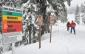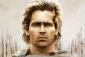5 posts / 0 newHello mga Engrs/ Sirs,

Pwede po ba patulong ng correct answer and solution for this question:

A series of square figures are made with match sticks. If the first three figures are the following, how many matchsticks will be needed to form the 6th figure? see link po, thank you in advance po.Jhun VertAng hirap naman... i-share natin to sa FB.meron ako possible answer, but mali pa rin...

Alexander1 square = 4 match sticks
4 squares = 12 match sticks
6 squares = 17 match sticksthanks alexander, but I am looking for the 6th figure, how many matchsticks are needed to figure out the 6th one?

• Mathematics inside the configured delimiters is rendered by MathJax. The default math delimiters are $$...$$ and $...$ for displayed mathematics, and $...$ and $...$ for in-line mathematics.